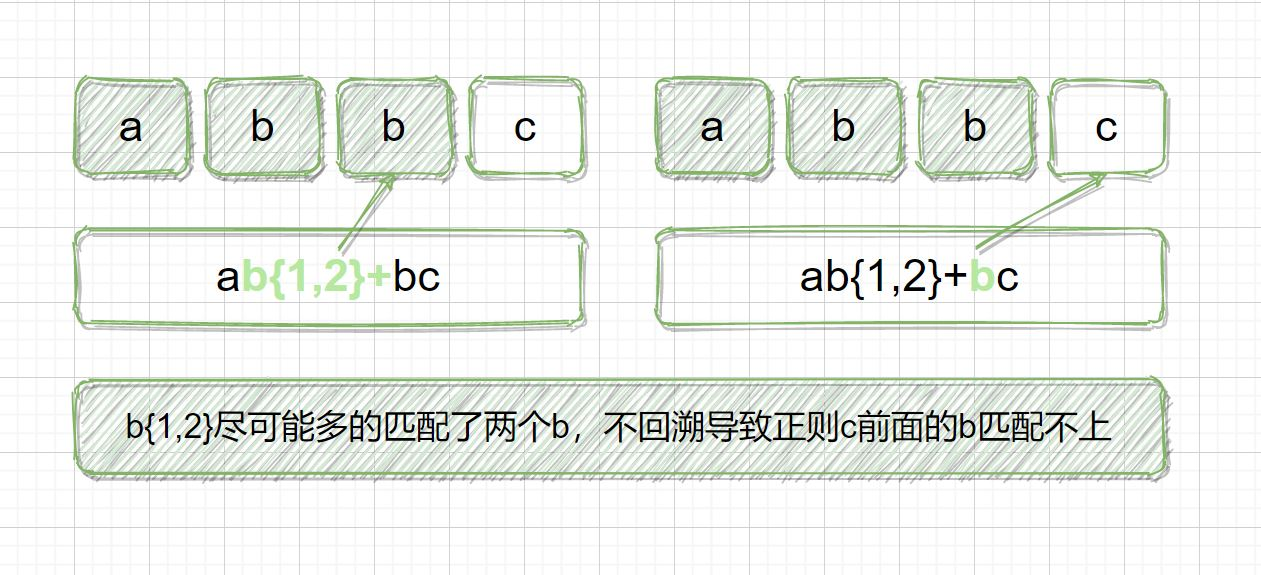# 正则表达式量词与贪婪的使用详解

更新时间：2021年07月26日 09:48:56   作者：容华谢后

## 1.量词* {0,} ab*

a 或者 abb
+ {1,} ab+

ab 或者 abb

? {0,1} ab?

## 2.贪婪模式前传

### 2.1 使用 a+ 进行匹配```import re

print(re.findall(r'a+', 'aaabb'))

```

### 2.2 使用 a* 进行匹配```import re

print(re.findall(r'a*', 'aaabb'))

```

## 3.贪婪模式

a* 在匹配字符串 aaabb 时，会尽可能多的把前面的 a 都匹配上，直到第一个字母 b 不满足要求为止，匹配上3个 a，后面每次匹配的都是空字符串。

## 4.非贪婪模式```import re

// 贪婪匹配
print(re.findall(r'a*', 'aaabb'))

// 非贪婪匹配
print(re.findall(r'a*?', 'aaabb'))

```## 5.独占模式

### 5.1 贪婪匹配过程```import regex

print(regex.findall(r'ab{1,3}c', 'abbc'))

```

### 5.2 非贪婪匹配过程```import regex

print(regex.findall(r'ab{1,3}?c', 'abbc'))

```

### 5.3 独占匹配过程```import regex

print(regex.findall(r'ab{1,2}+bc', 'abbc'))

```

## 6.写在最后